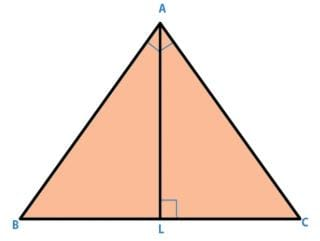In a triangle ABC,∠A = 900, AL is drawn p...

### Related TestIn a triangle ABC,∠ A = 900, AL is drawn perpendicular to BC, Then ∠ BAL is equal to:• a)
∠ALC
• b)
∠ACB
• c)
∠BAC
• d)
∠B - ∠BAL
• e)
None of theseANUSHA MARRI∠ BAL + ∠ B + 900 = 1800
or, ∠ BAL + ∠ B = 900
or, ∠ BAL = 900 - ∠ B ----------- (1)
Now, in Δ ABC,
∠ ACB + ∠ B + ∠ A = 1800
∠ ACB = 900
-∠ B ----- (2)
From, (1) and (2),
∠ BAL = ∠ ACB.View courses related to this question Explore GMAT courses
 Explore GMAT coursesView courses related to this question1 Crore+ students have signed up on EduRev. Have you?

• ### Two concentric circles have their centers at point O such that a line segme... more(Scan QR code)